# AP Calculus BC : Fundamental Theorem of Calculus with Definite Integrals

## Example Questions

2 Next →

### Example Question #11 : Fundamental Theorem Of Calculus And Techniques Of Antidifferentiation

Evaluate the following integral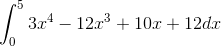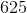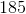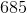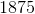Explanation:

Evaluate the following integralLet's begin by recalling our "reverse power rule" AKA, the antiderivative form of our power rule.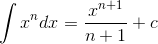In other words, all we need to do for each term is increase the exponent by 1 and then divide by that number.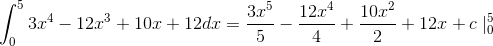Let's clean it up a little to get: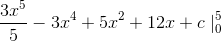Now, to evaluate our integral, we need to plug in 5 and 0 for x and find the difference between the values. In other words, if our integrated function is F(x), we need to find F(5)-F(0).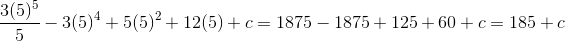Next, let's look at F(0). If you look at our function carefully, you will notice that F(0) will cancel out all of our terms except for +c. So, we have the following: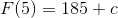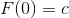Finding the difference cancels out the c's and leaves us with 185.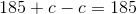2 Next →